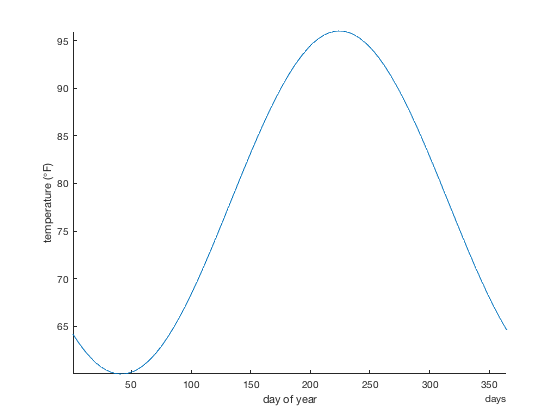# sineval documentation

sineval produces a sinusoid of specified amplitude and phase with a frequency of 1/yr.

For related functions, see sinefit and sinefit_bootstrap documentation.

Back to Climate Data Tools Contents

## Syntax

`y = sineval(ft,t)`

## Description

y = sineval(ft,t) evaluates a sinusoid of given fit parameters ft at times t. Times t can be in datenum, datetime, or datestr format, and parameters ft correspond to the outputs of the sinefit function and can have 2 to 5 elements, which describe the following:

• 2: ft = [A doy_max] where A is the amplitude of the sinusoid, and doy_max is the day of year corresponding to the maximum value of the sinusoid. The default TermOption is 2.
• 3: ft = [A doy_max C] also estimates C, a constant offset. Solving for adds processing time, so you may prefer to estimate C on your own simply as the mean of the input y. However, if you can't assume C=mean(y), you may prefer this three-term solution.
• 4: ft = [A doy_max C trend] also estimates a linear trend over the entire time series in units of y per year. Again, simultaneously solving for four terms will be much more computationally expensive than solving for two yerms, so you may prefer to estimate the trend on your own with polyfit, then calculate the two-term sine fit on your detrended data.
• 5: ft = [A doy_max C trend quadratic_term] also includes a quadratic term in the solution, but this is experimental for now, because fitting a polynomial to dates referenced to year zero tends to be scaled poorly.

## Example

I live in Austin, Texas, where the hottest day of the year occurs around August 12 at around 96 degrees F, and it's about 36 degrees colder in the winter. In ther words, the amplitude of the seasonal sinusoid might be 18 degrees, where the maximum value occurs on julian day 224, and the mean value is 78 degrees. Using these crude numbers, here's a year's climatology for Austin's daily high temperatures:

```% t can be just about any date format and it'll be okay:
t = days(1:365);

% Sinusoid of high temperatures:
HighTemp = sineval([18 224 78],t);

plot(t,HighTemp)
axis tight
box off
xlabel 'day of year'
ylabel 'temperature ({\circ}F)'
```## Author Info

This function is part of the Climate Data Toolbox for Matlab. The sinefit, sineval, and sinefit_bootstrap functions as well as the supporting documentation were written by Chad A. Greene of the University of Texas Institute for Geophysics.